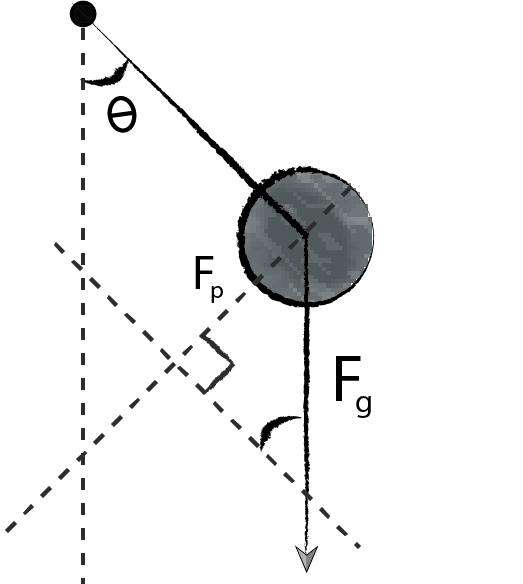Kanata Art center
HOME > MATHEMATICS > Physics

#Course Description

The objective of this course is to provide an in-depth presentation of Newtonian mechanics. Students that complete the course will be well prepared for a University/College level introductory physics course.

### Term One

• math skills required for physics: Cartesian Coordinates and vectors
• 1-dimensional kinematics
• math skills required for physics: trigonometric functions
• 2-dimensional kinematics
• math skills required for physics: quadratic equations
• applications of kinematics
• forces and Newton’s Laws of Motion
• applications of Newton’s Laws: incline planes
• applications of Newton’s Laws: pulleys
• applications of Newton’s Laws: friction and advanced problems

### Term Two

• review of term one material
• energy and work
• application of Energy and Work: springs and pendulums
• circular motion
• gravitation
• oscillations
• course review